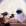Mathematicsuhfinn:

A substance decays so that the amount A of the substance left after t years is given by: A = A_0 · (0.9) ^ t , where A 0 is the original amount of the substance. What is the half-life (the amount of time that it takes to decay to half the original amount) of this substance rounded to the nearest tenth of a year? 5.6 years 6.1 years 6.6 years 7.5 yearsDuarteME:

As you can read in the question, the half-life is the time $$\tau$$ such that $$A(\tau) = \dfrac{A_0}{2}$$. Can you see how to proceed from here?kittybasil: \[\frac{3}{14}+\frac{10}{21}\div \left(\frac{3}{7}\right)\left(\frac{9}{4}\right)stephaniepargas12: reveal the following passages. how does the poet's use of alliteration influenceEsmeperez2003: determine the length of the object shownkainpate: Jerry deposits $5,000 at the end of each year in an account earning 2.25% interest, compounded annually. 41 minutes ago 0 Replies 0 Medalsidontcare369: Marjorie is trying to determine the identity of an unknown substance. After running tests, she determines that the substance is insoluble in CCl4 with a den 1 hour ago 1 Reply 0 Medalscburry: Which statement best describes the difference between the way the poets address t 1 hour ago 0 Replies 0 Medalskris062105: Find MN such that LN = 5.7. 1 hour ago 0 Replies 0 MedalsBlueStar: Hi, please show me how to solve this problem. A compound consists of carbon, hydrogen, oxygen and bromine. 1 hour ago 0 Replies 0 MedalsOlga11024: Name the kind or kinds of symmetry the following figure has: point, line, plane, or none. 2 hours ago 0 Replies 0 Medalsferdean: Blake Turner, a pharmacy technician, earns an annual gross pay of$41,300. He is married with two dependents, and his exemptions total \$8,000.